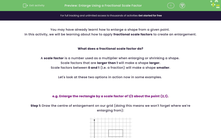# Enlarge Using a Fractional Scale Factor

In this worksheet, students will enlarge shapes using fractional and whole number scale factors, as well as finding scale factors and centres of enlargement once a scale factor has been applied.Key stage:  KS 4

Year:  GCSE

GCSE Subjects:   Maths

GCSE Boards:   AQA, Pearson Edexcel, Eduqas, OCR,

Curriculum topic:   Geometry and Measures, Basic Geometry, Congruence and Similarity

Popular topics:   Geometry worksheets

Difficulty level:#### Worksheet Overview

You may have already learnt how to enlarge a shape from a given point.

In this activity, we will be learning about how to apply fractional scale factors to create an enlargement.

What does a fractional scale factor do?

scale factor is a number used as a multiplier when enlarging or shrinking a shape.

Scale factors that are larger than 1 will make a shape larger.

Scale factors between 0 and 1 (i.e. a fraction) will make a shape smaller.

Let's look at these two options in action now in some examples.

e.g. Enlarge the rectangle by a scale factor of 1/2 about the point (2,1).

Step 1: Draw the centre of enlargement on our grid (doing this means we won't forget where we're enlarging from):Step 2: Find the movement from the centre to one of the points on the shape:We can see that to get to the bottom left corner of the rectangle, we move 2 squares to the right and 4 squares up.

Step 3: Multiply this movement by the scale factor.

Our scale factor is 1/2, so this movement, when halved, becomes: 1 right and 2 up.

Step 4: Plot this point on the diagram:When we are plotting this point, it is vital that we count from the centre of enlargement every time.

Step 5: Repeat steps 2 - 4 for the other corners of the rectangle:Step 6: Draw the enlarged shape and label the image and enlargement:And that's all there is to it - we have our new shape shown on our grid!

As the scale factor applied here was between 0 and 1, our rectangle has become smaller

When labelling our new shape, we tend to use an apostrophe (') after its original name, as shown above.

e.g. Shape a is enlarged to become shape a'. Find the scale factor and centre of enlargement for this change.Finding the scale factor

To find this, we simply need to compare one of the pairs of sides.

If we use the base of each triangle, shape a is 3 cm long and shape a' is 1 cm long.

This gives a scale factor of 1/3.

(Remember, as our second shape is smaller, our scale factor will be between 0 and 1.)

Finding the centre of enlargement

To find this, we need to join up two pairs of matching corners with straight lines and see where they cross, like this:We can see on our diagram above that the two lines cross at the point (1,0).

So (1,0) is our centre of enlargement.

In this activity, we will enlarge shapes using fractional and whole number scale factors, as well as finding scale factors and centres of enlargement once a scale factor has been applied.

If possible, you will want to have a pencil and squared paper handy to help you map these enlargements accurately. You may be asked to do this in an exam so it is definitely worth practising on paper now.

### What is EdPlace?

We're your National Curriculum aligned online education content provider helping each child succeed in English, maths and science from year 1 to GCSE. With an EdPlace account you’ll be able to track and measure progress, helping each child achieve their best. We build confidence and attainment by personalising each child’s learning at a level that suits them.

Get started#### Popular Maths topics

••••